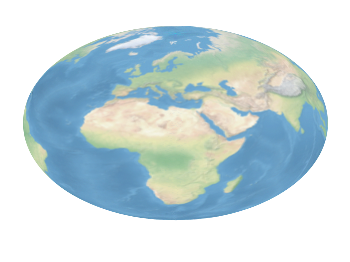# Gnuplotting

Create scientific plots using gnuplot

January 31st, 2014 | 3 Comments

And another plot of the world. This time we are dealing with the raster data from Natural Earth. This data is normally available as tif-files. To use them in gnuplot we have to convert them first, then we can create a plot as shown in Fig. 1.Fig. 1 Cross-blended hypsometric tint plot of the world. (code to produce this figure, data)

The conversion is done by the convert_natural_earth script. There the tif-file is first scaled down to the desired resolution using imagemagick. Afterwards it is converted to a text file and reordered for the `splot` command of gnuplot. The text file includes the longitude, latitude and three rgb color values.
You have to invoke the script in the following way.

```\$ ./convert_natural_earth \$RES \$FILE
```

where `\$RES` is the desired resolution in pixel of your gnuplot plot and `\$FILE` the input tif-file.
After finished we can plot the resulting text file simply by

```set datafile separator ','
set size ratio -1
plot 'HYP_50M_SR_W_350px.txt' w rgbimage
```

which results in Fig. 1.Fig. 2 Natural Earth II shaded relief and water plot of the world. (code to produce this figure, data)

The image can also be projected on a 3D figure of the world as shown in Fig. 2. To achieve this the three rgb values have to be summarized in one value and the `rgb variable` line color option has to be chosen together with `pm3d`.

```rgb(r,g,b) = 65536 * int(r) + 256 * int(g) + int(b)
set mapping spherical
set angles degrees
splot 'NE2_50M_SR_W_700px.txt' u 1:2:(1):(rgb(\$3,\$4,\$5)) w pm3d lc rgb variable
```